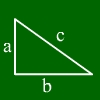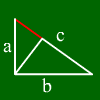# Pythagoras calculation

For rightangled triangles the following formulas hold:
t_2
a▓+b▓=c▓ (Pythagoras theorem)
a▓=c*p and b▓=c*q ( Euclids cathetus theorem)
h▓=p*q (Euclids height theorem)
sin alpha = a/c

## What is a rightangled triangle?A rightangled triangle is (as the name says) a triangle containing a right angle (i.e. a 90░-angle). This property makes calculations very easy.
The side opposing of the right angle is called hypotenuse, the other sides are called cathetus or legs.
In the example on the left the right angle is opposite of c. Therefore, c is the hypotenuse and a and b are the legs.

## What formulas hold for right angled triangles?

In the right angled triangle, the pythagoras theorem holds: a² +b² = c². This means that c equals the square root of (a² +b² ) or b equals the square root of (c² - a²).Further, for the parts of the hypotenuse (called p and q, p being below a and q being below b) those formulas hold:
a▓=c*p and b▓=c*q ( Euclids cathetus theorem)
A third formula is the height rule making the following statement about the height on c: h▓=p*q.
The area of a right-angled triangle is also easily computed cause it is simply equal to (leg * other leg)/2.

For further information, just move the mouse over one of the words below and the corresponding part of the triangle gets marked.Leg a Leg b Hypotenuse c
Hypotenuse part p Hypotenuse part q Area Height on c

## How to prove the Pythagorean theorem?

One possibility is shown by our flash animation:

Mathepower is a free Pythagoras calculator. E.g. you can compute by using the formula a▓+b▓=c▓ or other formulas.